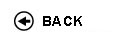Politecnico di Torino
Academic Year 2009/10
02BQYDR, 02BQYAX, 02BQYAY, 02BQYDS
Mathematical methods for engineering I
1st degree and Bachelor-level of the Bologna process in Mechanical Engineering - Vercelli
1st degree and Bachelor-level of the Bologna process in Civil Engineering - Vercelli
Master of science-level of the Bologna process in Civil Engineering - Vercelli
Espandi...
 Teacher Status SSD Les Ex Lab Tut Years teaching Ferrarotti MassimoAC MAT/03 40 30 0 0 9
 SSD CFU Activities Area context MAT/05 7 D - A scelta dello studente A scelta dello studente
 Objectives of the course The goal of this course is to learn multivariate calculus which is fundamental for engineering applications. Expected skills The student will be able to deal with elementary analytic geometry in the space, study multivariate functions and to compute integrals both on curves or surfaces and on multivariate domains. Prerequisites The students are expected to know results and techniques from calculus in one variable, linear algebra (matrix, vector spaces, eigenvalues and quadratic forms, trigonometry and plane geometry. Syllabus - Elements of space geometry: lines, planes , plane space curves, conics, surfaces, quadrics, cones and - cylinders. - Parametric space curves, tangent line and length of a curve. Integration along a curve. - Topology of real n-dimensional spaces; continuity of multivariate functions, gradients and directional derivatives. Maxima and Minima, constrained Extrema and Lagrange multipliers. - Parametric surfaces, rotational surfaces, graphs of two variable functions, plane and spatial regular transformations. - Vector fields, rotational fields. Integrals on curves, exact differentials. - Multiple integration: double and triple integrals. Integrals in polar, cylindrical and spherical coordinates. Gauss-Green formula and Stoke's Theorem. Laboratories and/or exercises Exercise sections are done during the course. Bibliography S.Greco, P.Valabrega, Lezioni di Geometria, ed.Levrotto e Bella. A.Sanini, Elementi di Geometria, ed.Levrotto e Bella. M.Bramanti, C.D.Pagani, S.Salsa, MATEMATICA, calcolo infinitesimale e algebra lineare, ed. Zanichelli. S.Salsa, A.Squellati, ESERCIZI DI MATEMATICA, calcolo infinitesimale e algebra lineare Vol.2, , ed. Zanichelli. Fusco N., Marcellini P., Sbordone C., Elementi di Analisi Matematica II, ed. Liguori. Revisions / Exam The examination consists in a written proof based on resolution of exercises. No calculator, no texts or notes can be used during the examination. Programma definitivo per l'A.A.2009/10© Politecnico di Torino
Corso Duca degli Abruzzi, 24 - 10129 Torino, ITALY